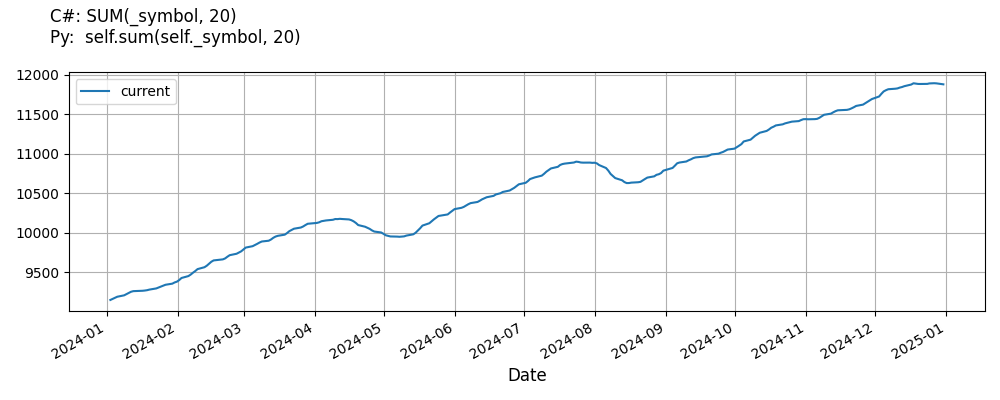# Supported Indicators

## Sum

### Introduction

This indicator represents an indicator capable of tracking the sum for the given period

To view the implementation of this indicator, see the LEAN GitHub repository.

### Using SUM Indicator

To create an automatic indicators for Sum, call the SUM helper method from the QCAlgorithm class. The SUM method creates a Sum object, hooks it up for automatic updates, and returns it so you can used it in your algorithm. In most cases, you should call the helper method in the Initialize method.

public class SumAlgorithm : QCAlgorithm
{
private Symbol _symbol;
private Sum _sum;

public override void Initialize()
{
_sum = SUM(_symbol, 20);
}

public override void OnData(Slice data)
{
{
// The current value of _sum is represented by itself (_sum)
// or _sum.Current.Value
Plot("Sum", "sum", _sum);

}
}
}
class SumAlgorithm(QCAlgorithm):
def Initialize(self) -> None:
self.sum = self.SUM(self.symbol, 20)

def OnData(self, slice: Slice) -> None:
# The current value of self.sum is represented by self.sum.Current.Value
self.Plot("Sum", "sum", self.sum.Current.Value)



The following reference table describes the SUM method:

### SUM()1/1

            Sum QuantConnect.Algorithm.QCAlgorithm.SUM (
Symbol                           symbol,
Int32                            period,
*Nullable<Resolution>      resolution,
*Func<IBaseData, Decimal>  selector
)


Creates a new Sum indicator.

If you don't provide a resolution, it defaults to the security resolution. If you provide a resolution, it must be greater than or equal to the resolution of the security. For instance, if you subscribe to hourly data for a security, you should update its indicator with data that spans 1 hour or longer.

You can manually create a Sum indicator, so it doesn't automatically update. Manual indicators let you update their values with any data you choose.

Updating your indicator manually enables you to control when the indicator is updated and what data you use to update it. To manually update the indicator, call the Update method with time/number pair, or an IndicatorDataPoint. The indicator will only be ready after you prime it with enough data.

public class SumAlgorithm : QCAlgorithm
{
private Symbol _symbol;
private Sum _sum;

public override void Initialize()
{
_sum = new Sum(20);
}

public override void OnData(Slice data)
{
if (data.Bars.TryGeValue(_symbol, out var bar))
{
_sum.Update(bar.EndTime, bar.Close);
}

{
// The current value of _sum is represented by itself (_sum)
// or _sum.Current.Value
Plot("Sum", "sum", _sum);

}
}
}
class SumAlgorithm(QCAlgorithm):
def Initialize(self) -> None:
self.sum = Sum(20)

def OnData(self, slice: Slice) -> None:
bar = slice.Bars.get(self.symbol)
if bar:
self.sum.Update(bar.EndTime, bar.Close)

# The current value of self.sum is represented by self.sum.Current.Value
self.Plot("Sum", "sum", self.sum.Current.Value)



To register a manual indicator for automatic updates with the security data, call the RegisterIndicator method.

public class SumAlgorithm : QCAlgorithm
{
private Symbol _symbol;
private Sum _sum;

public override void Initialize()
{
_sum = new Sum(20);
RegisterIndicator(_symbol, _sum, Resolution.Daily);
}

public override void OnData(Slice data)
{
{
// The current value of _sum is represented by itself (_sum)
// or _sum.Current.Value
Plot("Sum", "sum", _sum);

}
}
}
class SumAlgorithm(QCAlgorithm):
def Initialize(self) -> None:
self.sum = Sum(20)
self.RegisterIndicator(self.symbol, self.sum, Resolution.Daily)

def OnData(self, slice: Slice) -> None:
# The current value of self.sum is represented by self.sum.Current.Value
self.Plot("Sum", "sum", self.sum.Current.Value)



The following reference table describes the Sum constructor:

### Sum()1/2

            Sum QuantConnect.Indicators.Sum (
string  name,
int     period
)


Initializes a new instance of the Sum class with the specified name and period.

### Sum()2/2

            Sum QuantConnect.Indicators.Sum (
int  period
)


Initializes a new instance of the Sum class with the default name and period.

### Visualization

The following image shows plot values of selected properties of Sum using the plotly library.You can also see our Videos. You can also get in touch with us via Discord.